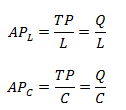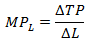# Concept of production

1. Total production (TP):
Total production refers to total quantity of output produced by using both fixed and variable factors of production but in short run production is only the function of variable factor.
TP = F(Ql)
Where,
TP = total production
F = functional relationship
Ql = quantity of labor

2. Average production (AP):
Average production of a factor which is obtained by dividing the total production, by number of input used in production or it is the per unit output produced by factors used.Where,
APL = average product of labor
APC = average product of capital
TP = total product
L = no. of labors
C = capital

3. Marginal production (MP):
Marginal production of an input is the change in TP due to change in an additional unit of input in production. Mathematically it is expressed asWhere,
MPL = marginal production of labor
∆TP = change in total product
∆L = change in no. of labor